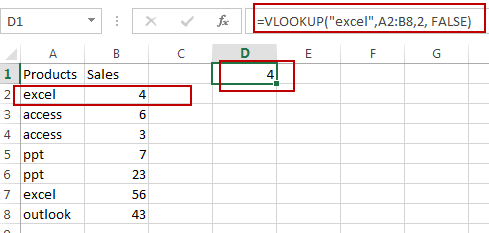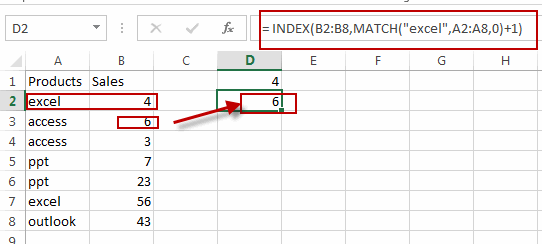# How to Look up Value and Return Adjacent Cell Value in Excel

This post will guide you how to lookup a value from a range of cells and return match from adjacent cell in Excel.  How do I Vlookup a value and return a value in corresponding, adjacent cell in Excel.

## Look up Value and Return Adjacent Cell Value

Assuming that you have a list of data in range A1:B8, and you need to vlookup one product name and return the adjacent sale value of Sales column. And you can use a formula based on the VLOOKUP function. Like this:

`=VLOOKUP("excel",A2:B8,2, FALSE)`

Type this formula in a blank cell and then press Enter key to apply it.

The adjacent cell value of product  “excel” in Column Product is returned in Cell D1.If you want to lookup value and return the value in the next cell of the adjacent cell, you can use another formula based on the INDEX function and the MATCH function. Like this:

`= INDEX(B2:B8,MATCH(“excel”,A2:A8,0)+1)`

Type this formula in a blank cell and then press Enter key to apply it.### Related Functions

• Excel VLOOKUP function
The Excel VLOOKUP function lookup a value in the first column of the table and return the value in the same row based on index_num position.The syntax of the VLOOKUP function is as below:= VLOOKUP (lookup_value, table_array, column_index_num,[range_lookup])….
• Excel INDEX function
The Excel INDEX function returns a value from a table based on the index (row number and column number)The INDEX function is a build-in function in Microsoft Excel and it is categorized as a Lookup and Reference Function.The syntax of the INDEX function is as below:= INDEX (array, row_num,[column_num])…
• Excel MATCH function
The Excel MATCH function search a value in an array and returns the position of that item.The syntax of the MATCH function is as below:= MATCH  (lookup_value, lookup_array, [match_type])….

Sidebar